# C++ program to print the Fibonacci series

In this article, you will learn and get code to print Fibonacci series using a C++ program in the following ways:

In the first program, if the user enters a number, say 10, as input, then the program prints the Fibonacci series with 10 terms like:

`0  1  1  2  3  5  8  13  21  34`

Whereas in the second program, if the user enters 10 as input, then the program prints a Fibonacci series in which the last number can not be greater than 10. Here is the Fibonacci series up to 10:

`0  1  1  2  3  5  8`

In the third program, we've implemented both features using switch cases and user-defined functions.

But before going through these programs, let's understand the Fibonacci series.

### What is the Fibonacci series?

The Fibonacci series is a sequence of numbers in which the first two numbers start with 0 and 1. And all the upcoming numbers get calculated as the sum of their previous two numbers in the following ways:

• 0 as the first number
• 1 as the second number
• The third number is 1. The third number is the sum of the first (0) and second (1) numbers. That is, 1+0.
• The fourth number is 2. The fourth number is the sum of the third (1) and second (1) numbers. That is, 1+1.
• The fifth number is 3. That is, 2+1.
• The sixth number is 5. That is, 3+2.
• and so on.

## Print the Fibonacci Series of N Terms

To print a Fibonacci series of N terms in C++ programming, you have to ask the user to enter the size of the Fibonacci sequence or series. Then, as shown in the program below, generate and print a Fibonacci series of the given size:

```#include<iostream>
using namespace std;
int main()
{
int a=0, b=1, c, tot, temp, i;
cout<<"Enter the Size of Fibonacci Sequence: ";
cin>>tot;
cout<<"\nFibonacci Series of "<<tot<<" Terms:\n";
for(i=1; i<=tot; i++)
{
if(i==1)
c = 0;
else if(i==2 || i==3)
c = 1;
else
{
temp = a;
a = b;
b = c;
c = a+b;
}
if(i==tot)
cout<<c;
else
cout<<c<<", ";
}
cout<<endl;
return 0;
}```

This program was built and runs under the Code::Blocks IDE. Here is its sample run:Now supply the input as a number to print the Fibonacci series. Here the number indicates the number of digits or terms (whatever you say) the Fibonacci series contains. For example, if the user enters 10 as input, then the program prints a Fibonacci series that contains 10 digits or numbers. Here is the sample output with user input: 10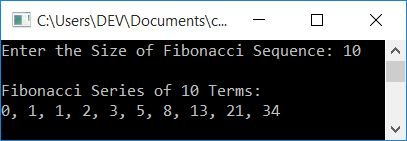## Print the Fibonacci Series up to the given number

This program generates Fibonacci numbers in such a way that the last number can't be greater than the number entered by the user, as shown in the program given below.

The question is: write a program in C++ to print Fibonacci numbers up to a given number. Here is its answer:

```#include<iostream>
using namespace std;
int main()
{
int a=0, b=1, c, limit, temp, i;
cout<<"Enter the Limit: ";
cin>>limit;
cout<<"\nFibonacci Series upto "<<limit<<":\n";
for(i=1; ; i++)
{
if(i==1)
c = 0;
else if(i==2 || i==3)
c = 1;
else
{
temp = a;
a = b;
b = c;
c = a+b;
}
if(c>limit)
break;
cout<<c<<"  ";
}
cout<<endl;
return 0;
}```

Here is its sample run with user input, 200: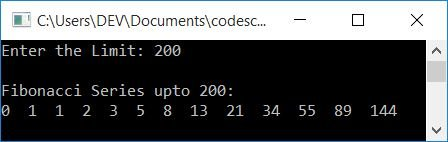## Fibonacci Series using Function

This program generates and prints Fibonacci series of N terms and up to a given number for both, depending on what the user chooses from the menu-driven feature. This program prints the Fibonacci series in both ways using user-defined functions FiboOfNTerm() and FiboUptoGivenNumber().

```#include<iostream>
using namespace std;
void FiboOfNTerm(int);
void FiboUptoGivenNumber(int);
int main()
{
int ch, N, limit;
do
{
cout<<"1. Fibonacci Series of N Term\n";
cout<<"2. Fibonacci Series upto Given Number\n";
cout<<"3. Exit\n";
cin>>ch;
switch(ch)
{
case 1:
cout<<"\nEnter the Value of N: ";
cin>>N;
FiboOfNTerm(N);
break;
case 2:
cout<<"\nEnter the Number (Limit): ";
cin>>limit;
FiboUptoGivenNumber(limit);
break;
case 3:
return 0;
default:
cout<<"\nWrong Input!";
break;
}
cout<<"\n\n";
}while(ch==1 || ch==2);
cout<<endl;
return 0;
}
void FiboOfNTerm(int tot)
{
int i, a=0, b=1, c, temp;
for(i=1; i<=tot; i++)
{
if(i==1)
c = 0;
else if(i==2 || i==3)
c = 1;
else
{
temp = a;
a = b;
b = c;
c = a+b;
}
cout<<c<<"  ";
}
}
void FiboUptoGivenNumber(int limit)
{
int i, a=0, b=1, c, temp;
for(i=1; ; i++)
{
if(i==1)
c = 0;
else if(i==2 || i==3)
c = 1;
else
{
temp = a;
a = b;
b = c;
c = a+b;
}
if(c>limit)
break;
cout<<c<<"  ";
}
}```

Here is its sample run. This is the initial output: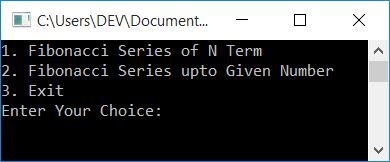Now enter your choice, that is, in what way you want to print the Fibonacci series. Here is the sample output produced after entering 1 as a choice and pressing the ENTER key: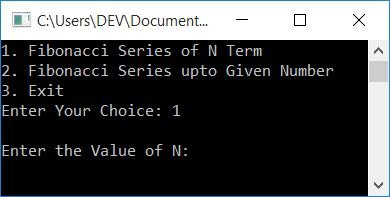Enter the value of N to generate and print the Fibonacci series of N numbers. Here is its sample output after supplying or entering 10 as the value of N: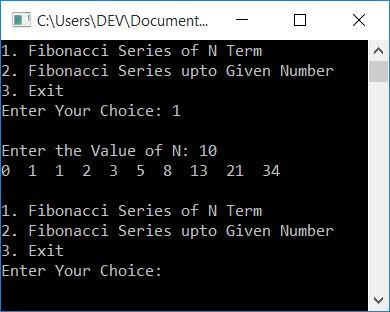As you can see, the Fibonacci series of 10 terms gets printed, and the choice again gets displayed to continue the operation. Let's check it out with the second and then third option, as shown in the snapshot given below: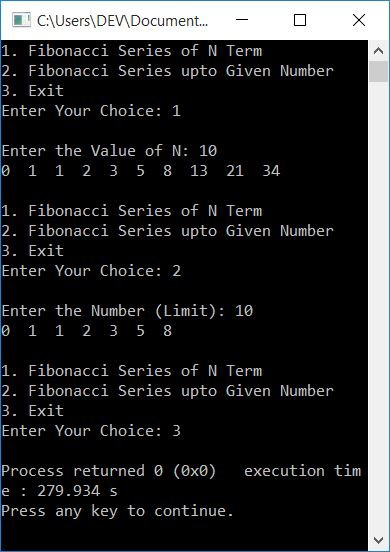#### The same program in different languages

C++ Quiz

« Previous Program Next Program »